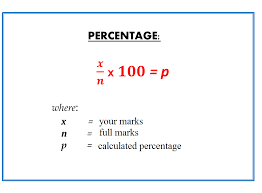# How to calculate percentage of marks?When I was in school calculating my exam marks per cent was my first task after my examination. Every student of colleges or schools wants to know his percentage of marks so that he or she can know what division they got in 10th, 12th board exam or any other college or university exam. Calculation of marks percentage sometimes becomes confusing, especially for a student who is not good at mathematics. Here I am giving you a step-by-step method of calculating the percentage of obtained marks in a particular exam.

## What is a percentage?

A percentage is nothing but a fraction ratio of any number. It is denoted by the symbol % (Percent). Let’s make it more simple, If 100 is a number then 50 of a hundred (50/100) is called the 50% of 100. If we change the number from 100 to 1000 then the 50 Percent of 1000 will be 5000, which is also half of the number 1000. If we divide the number 1000 by 4 we will get 25% of the number 1000. In marks percentage calculation you can assume the Total of each paper’s marks as 100% and then you can easily calculate your percentage of marks without using any formula.  Suppose the total marks of each subject is 400 and you obtain a total of 300 marks after adding all paper numbers.  So here 400 is now 100%, 200 is now 50% and 40 is 10%.  Now let’s calculate the total 50% (for 200 marks)+20% (for 80 marks)+5% (for remaining 20 marks). Now add all your marks which are now become 300 and add all your per cent marks which are now become 75%.  This is the simplest way of doing percentages calculation in a fraction manner.

## The formula for calculating the percentage of any number

Here is the simple formula for calculating the per cent of a number. You can use this formula to calculate the percentage of any number.

Formula:

Percentage, % = (Value / Total Value) × 100

## How to calculate the % of marks obtained in board exam

In each class students wants to calculate their exam marks per cent. The calculation depends on how many subjects marks you are adding to get your final result. In some classes like CBSE 10th board class or CBSE, 12th board class subject numbers may vary from stream to stream. Some students may have only 5 subjects while some may have 6 subjects.

Steps to calculate the percentage of marks

1. Add all the obtained marks and divide it by the maximum marks of the exam test.
2. multiple this number with 100 and you will get the desired per cent.

For example to calculate the per cent of the mark

Let a student obtain total of 540 marks in 6 different subjects out of 600 maximum marks. Then the percent will be calculated like: % of marks = (540/600)*100

% of marks = 90%Q.1 What is the formula for calculating percentage?

Answer: The formula to calculate the per cent is

P% =( X/Y)*100

Where  Y is the Total number, X is the achieved number and P is the final result in Percent.

Q.2 How to calculate the total percentage of marks?

Answer: For calculating your total percentage of marks you can divide the total obtained marks by the total of maximum marks of each subject. Now multiply the result by 100 to get the per cent.

Q.3 How do you find the percentage of 6 subjects?

Answer: To find the percentage of 6 subjects’ marks, just add all six subjects’ obtain marks, divide it by the total maximum marks of all six subjects and then multiply the final number with 100

Q.4 How is the 12th Class Mark percentage calculated?

Answer: You can follow the same steps given above to calculate the 12th board exam marks percentages easily.